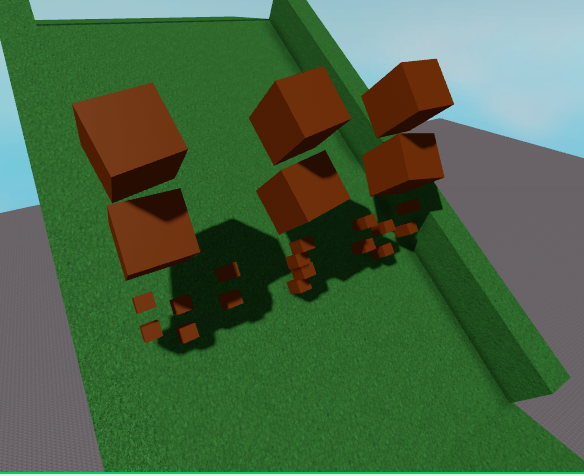# Level Randomizer Glitch

I’m trying to make a level randomizer that moves the level sections to predetermined Vector3 positions.

My code
``````local toplevel = math.floor(math.random(1,6))
print(toplevel)
local secondlevel = math.floor(math.random(1,6))
if secondlevel == toplevel then
repeat
secondlevel = math.floor(math.random(1,6))
print("It is!")
wait(0.01)
until secondlevel ~= toplevel
end
print(secondlevel)
local thirdlevel = math.floor(math.random(1,6))
if thirdlevel == toplevel or thirdlevel == secondlevel then
repeat
thirdlevel = math.floor(math.random(1,6))
print("It is!")
wait(0.01)
until thirdlevel ~= toplevel and thirdlevel ~= secondlevel
end
print(thirdlevel)
local fourthlevel = math.floor(math.random(1,6))
if fourthlevel == toplevel or fourthlevel == secondlevel or fourthlevel == thirdlevel then
repeat
fourthlevel = math.floor(math.random(1,6))
wait(0.01)
print("It is!")
until fourthlevel ~= toplevel and fourthlevel ~= secondlevel and fourthlevel ~= thirdlevel
end
print(fourthlevel)
local fifthlevel = math.floor(math.random(1, 6))
if fifthlevel == toplevel or fifthlevel == secondlevel or fifthlevel == thirdlevel or fifthlevel == fourthlevel then
repeat
fifthlevel = math.floor(math.random(1,6))
print("It is!")
wait(0.01)
until fifthlevel ~= toplevel and fifthlevel ~= secondlevel and fifthlevel ~= thirdlevel and fifthlevel ~= fourthlevel
end
print(fifthlevel)
local bottomlevel = math.floor(math.random(1,6))
if bottomlevel == toplevel or bottomlevel == secondlevel or bottomlevel == thirdlevel or bottomlevel == fourthlevel or bottomlevel == fifthlevel then
repeat
bottomlevel = math.floor(math.random(1,6))
print("It is!")
wait(0.01)
until bottomlevel ~= toplevel and bottomlevel ~= secondlevel and bottomlevel ~= thirdlevel and bottomlevel ~= fourthlevel and bottomlevel ~= fifthlevel
end
print(bottomlevel)

for i, v in ipairs(workspace.PinballFallLevelRandomizer:GetChildren()) do
for j, k in ipairs(v:GetChildren()) do
if k:IsA("BasePart") then
k.CanCollide = false
end
end
print(v.Name)
v:MoveTo(v[tonumber(toplevel)].Value)
print(v.PrimaryPart.Position)
end
``````
The result

The result:If anyone knows why this is happening, please let me know!

EDIT: BIG OOF. I THINK I FIXED I MYSELF: IT’S MOVING TO ONLY THE “topnumber” LEVEL, NONE OF THE OTHERS. SORRY GUYS

1 Like

Hard for me to read the code :v
A little hardcoded too, I see ur picture and I dont see any problem, what are u trying to achieve?

1 Like

in the loop at the end, change this

``````v:MoveTo(v[tonumber(toplevel)].Value)
``````

for this

``````v:MoveTo(v[tostring(toplevel)].Value)
``````

I do not usually code but I believe it’s the “1,6.” Have you tried changing it.